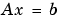MATLAB Function Referenceinv

Matrix inverse

Syntax

• Y = inv(X)

Description

Y = inv(X) returns the inverse of the square matrix X. A warning message is printed if X is badly scaled or nearly singular.

In practice, it is seldom necessary to form the explicit inverse of a matrix. A frequent misuse of inv arises when solving the system of linear equations. One way to solve this is with x = inv(A)*b. A better way, from both an execution time and numerical accuracy standpoint, is to use the matrix division operator x = A\b. This produces the solution using Gaussian elimination, without forming the inverse. See \ and / for further information.

Examples

Here is an example demonstrating the difference between solving a linear system by inverting the matrix with inv(A)*b and solving it directly with A\b. A random matrix A of order 500 is constructed so that its condition number, cond(A), is 1.e10, and its norm, norm(A), is 1. The exact solution x is a random vector of length 500 and the right-hand side is b = A*x. Thus the system of linear equations is badly conditioned, but consistent.

On a 300 MHz, laptop computer the statements

• n = 500;
Q = orth(randn(n,n));
d = logspace(0,-10,n);
A = Q*diag(d)*Q';
x = randn(n,1);
b = A*x;
tic, y = inv(A)*b; toc
err = norm(y-x)
res = norm(A*y-b)

produce

• elapsed_time =
1.4320
err =
7.3260e-006
res =
4.7511e-007

while the statements

• tic, z = A\b, toc
err = norm(z-x)
res = norm(A*z-b)

produce

• elapsed_time =
0.6410
err =
7.1209e-006
res =
4.4509e-015

It takes almost two and one half times as long to compute the solution with y = inv(A)*b as with z = A\b. Both produce computed solutions with about the same error, 1.e-6, reflecting the condition number of the matrix. But the size of the residuals, obtained by plugging the computed solution back into the original equations, differs by several orders of magnitude. The direct solution produces residuals on the order of the machine accuracy, even though the system is badly conditioned.

The behavior of this example is typical. Using A\b instead of inv(A)*b is two to three times as fast and produces residuals on the order of machine accuracy, relative to the magnitude of the data.

Algorithm

Inputs of Type Double

For inputs of type double, inv uses the following LAPACK routines to compute the matrix inverse:

 Matrix Routine Real DLANGE, DGETRF, DGECON, DGETRI Complex ZLANGE, ZGETRF, ZGECON, ZGETRI

Inputs of Type Single

For inputs of type single, inv uses the following LAPACK routines to compute the matrix inverse:

 Matrix Routine Real SLANGE, SGETRF, SGECON, SGETRI Complex CLANGE, CGETRF, CGECON, CGETRI